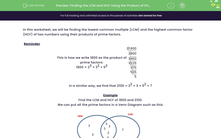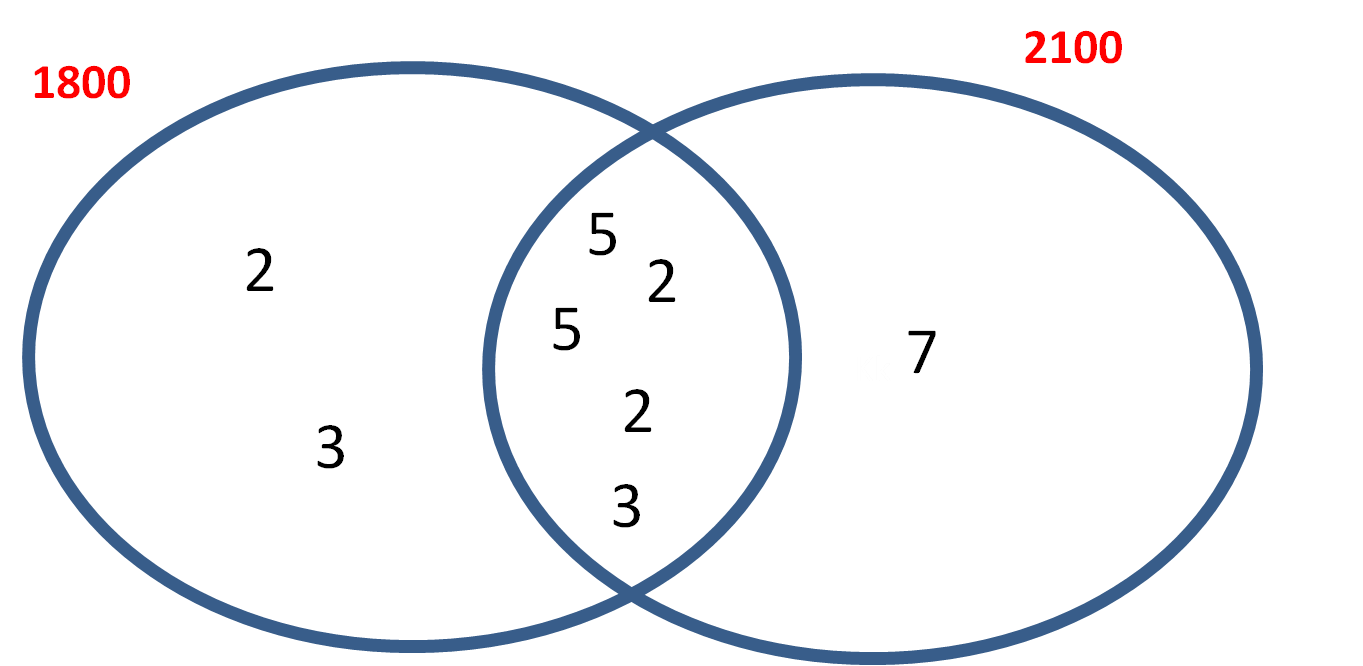# Finding the LCM and HCF Using the Product of Prime Factors

In this worksheet, students find the lowest common multiple and highest common factor using the product of prime factors.Key stage:  KS 3

Curriculum topic:   Number

Curriculum subtopic:   Use Concepts and Vocabulary for All Numbers

Difficulty level:#### Worksheet Overview

In this worksheet, we will be finding the lowest common multiple (LCM) and the highest common factor (HCF) of two numbers using their products of prime factors.

Reminder

 This is how we write 1800 as the product of prime factors: 1800 = 23 × 32 × 52 2 1 8 0 0 2 9 0 0 2 4 5 0 3 2 2 5 3 7 5 5 2 5 5

In a similar way, we find that 2100 = 22 × 3 × 52 × 7

Example

Find the LCM and HCF of 1800 and 2100.

We can put all the prime factors in a Venn Diagram such as this:To find the HCF, we multiply together the numbers in the intersection.

HCF = 2 × 2 × 5 × 5 × 3 = 300

To find the LCM, we multiply all the numbers in the Venn Diagram

LCM = 2 × 2 × 2 × 3 × 3 × 5 × 5 × 7 = 1800 × 7 = 12600

### What is EdPlace?

We're your National Curriculum aligned online education content provider helping each child succeed in English, maths and science from year 1 to GCSE. With an EdPlace account you’ll be able to track and measure progress, helping each child achieve their best. We build confidence and attainment by personalising each child’s learning at a level that suits them.

Get started